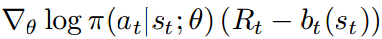# What's the right way of implementing policy gradient?

Hello everyone!
I’m trying to perform this gradient update directly, without computing loss.But I simply haven’t seen any ways I can achieve this. Looks like first I need some function to compute the gradient of policy, and then somehow feed it to the backward function.

1 Like

I think you should look at this topic explaining the tensor.reinforce method:

If the action is the result of a sampling, calling `action.reinforce(r)` acts as a policy gradient.
You can find a code example of implementation here:

Or you can just have
loss = samples_logprob * (reward - baseline)

(logprob is the output of the network, and reward and baseline are leaf variables)

If you do backward, the gradient backpropogated is equivalent to the policy gradient.

1 Like

hi everyone, I am new to pytorch, my question is a litter different from @Eddie_Li,

is there any way to change grad after backward?
I mean loss = right side of the red line
After backward, can I do pai(theta) * loss.grad, and then optimizer.step() ?
Is that way work?

Your loss would be something like

``````loss = - torch.sum(torch.log( policy(state) * (reward - baseline)))
``````

then you compute the gradient of this loss with respect to all the parameters/variables that requires a gradient in your code by calling:

``````loss.backward()
``````

and if you created, before the training loop, an optimizer associated to your policy like this:

``````optim_policy = optim.MyOptimizer( policy.parameters(), lr = wathever)
``````

you can update your optimizer simply like this, after each backward call of your loss:

``````optim_policy.step()
``````

1 Like

Thanks for your help, but my question is a little different
In my formula A* is a set of all possible episodes
when I update parameters , I want something like
or in other words
(p1loss(p1) + …+pNloss(pN)) , where p1+…+pN = 1
(loss(p1)+…+loss(pN)) / N
I want to change grad between loss.backward() and optim_policy.step()
I tried loss.grad() but it is NoneThen, I am not sure to understand your question… You want to use the policy as weights for the gradient update ? that way, you should re-write your loss:

``````weighted_loss = p1*loss(p1) + .. + pn*loss(pn)
weighted_loss.backward()
optimizer.step()
``````

Emm, here is the full formula
policy is the weight of loss.grad, not the weight of loss itself.

policy is the weight of loss.grad, not the weight of loss itself.

taken as a scalar quantity (that’s what I mean by weight) it’s just the same: `grad(w*x) = w*grad(x)`
you just have to make sure you are not using it as a variable of the tree (using pi.detach() should do it)

Thanks a lot, it looks like detach() is what I want:blush:

1 Like

I am doing a similar example to this and I am having trouble defining the “baseline” variable. I tried

``````base_line=Variable(Variable(torch.ones(2), requires_grad=True))
base_line=base_line
``````

and get the error “RuntimeError: element 0 of tensors does not require grad and does not have a grad_fn”. I only have one baseline value, could you suggest a way to define this varieble?

Generally, the baseline is an approximation of the expected reward, that does not depend on the policy parameters (so it does not affect the direction of the gradient).

This approximation can be the output of another network that takes the state as input and returns a value, and you minimize the distance between the observed rewards and the predicted values. Then, over a rollout, you predict the values of all states with this model, and remove the values from the rewards:

``````# policy = Policy() # the network that computes the prob of your actions
# value = Value() # the network that predicts the rewards
loss = - torch.sum( torch.log(policy(states)) * (rewards - values(states) )
``````

That’s the idea of the actor critic for policy gradient. You have a full example here: https://github.com/pytorch/examples/blob/master/reinforcement_learning/actor_critic.py

If your rollout is long enough, the sum of rewards approximates Q and the sum of values approximates V, so the term (rewards - values) approximate the advantage (Q-V) which is a non-biased estimator of the temporal difference (hence the name advantage-actor-critic).

1 Like

Just a quick question. The log probabilities are negative. If the rewards are positive, then the product with high rewards will make negative log prob values even lower (and loss even bigger)? Isn’t it opposite to what we need? Or the rewards should be negative? Did I miss anything?

the `log_prob*advantage` quantity is the objective to maximize. If a reward is negative, then having a large negative log_probs gives a large objective. At the opposite, if a reward is positive, then having small negative log_probs (close to 0) maximizes the objective.

With Pytorch, we need a loss to minimize, so we take `loss=-objective`.

Hello, I implemented the loss like you said, and it works.
However, why doesn’t it have the opposite sign? Because the given gradient is actually for gradient ascent.

@alexis-jacq I think your line is wrong

`loss = - torch.sum(torch.log( policy(state) * (reward - baseline)))`

Shouldn’t the `reward - baseline` be outside the `log(policy(state))`?

1 Like

I am thinking the same thing… if reward - baseline = 0 then this will give log(0)